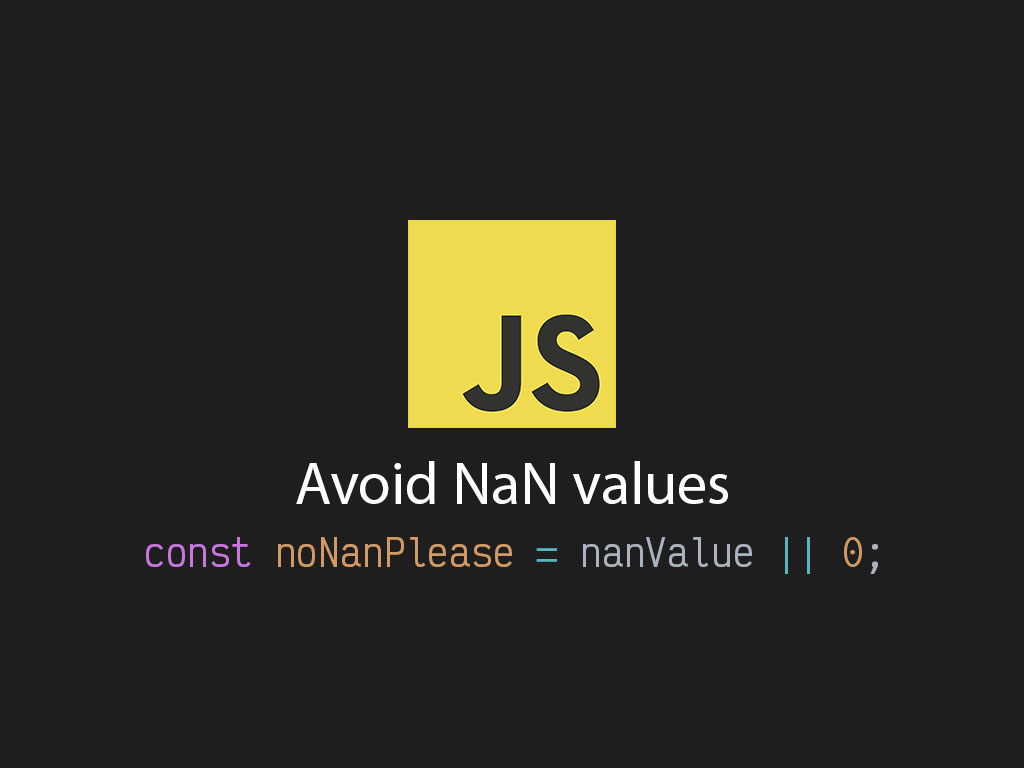# How to avoid NaN in JavaScriptAre you getting a `NaN` errors?

Here are 4 methods to avoid `NaN` values.

## Avoid #1: Mathematical operations with non-numeric string values

In JavaScript you can execute mathematical operations with numeric string values.

``````
// Subtracting
const a = "10" - "10"; // 0

// Multiply
const b = "10" * "10"; // 100

// Division
const c = "10" / "10" // 1
``````

But what if the string value is non-numeric?

``````
const y = 5 * "Apples"; // NaN
``````

Than `NaN` gets produced.

To avoid it completely, it’s best if you do all your mathematical operations with numbers.

Sometimes stringed values may come back from an API, just check if the value is `NaN` in the first place.

``````
isNaN("5") || 0
``````

## Avoid #2: Mathematical operations with functions

Doing mathematical operations with a function will result in a `NaN` value.

``````
function fooBar() {
// ...stuff
}

fooBar * 5 // NaN
``````

## Avoid #3: Mathematical operations with objects

Doing mathematical operations with a JavaScript object will result in a `NaN` value.

``````
const obj = {};

obj * 5 // NaN
``````

## Avoid #4: Mathematical operations with falsy values

Avoid doing mathematical operations with falsy values such as:

• `undefined`
• `NaN`
• `null`
• `false`
• empty string (`""`)

Just avoid it.

``````
const a = undefined + 5; // NaN

const b = NaN / 5; // NaN

const c = null - 5; // -5. Null behaves like a 0.

const d = false * 5; // -5. False behaves like a 0.

const e = "" + 10; // 10. Empty string behaves like a 0.
``````

## Conclusion

Stick to doing mathematical operations with number type values.

Here’s a fallback option that will convert your falsy value to 0.

``````
const a = nanValue || 0; // Converts false to value 0
``````

I like to tweet about JavaScript and post helpful code snippets. Follow me there if you would like some too!